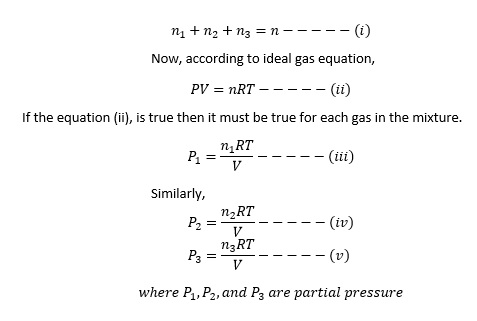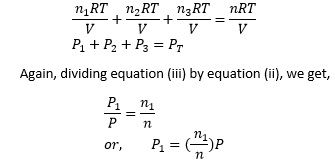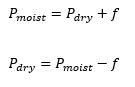# Dalton’s Law Of Partial Pressure

Let us consider a mixture of two non-reactive gases A and B enclosed in a container whose volume is V. Let the temperature be T. If by any means we can remove one of the component gas from the mixture then the pressure inside the container at that time is known as the partial pressure of the gas present in the container.
Partial pressure of a gas in a mixture of gases can be defined as that pressure which the gases would exert, if present alone in the container.
Statement of Dalton’s Law: The total pressure of a mixture of gases is equal to the sum of partial pressure of the component gases present in the mixture at constant temperature.
If P1, P2 and P3 are the partial pressure of three non-reacting gases enclosed in a container whose volume is V. Then according to Dalton’s Law, the total pressure is equal to sum of partial pressure.
Mathematical deduction of Dalton’s Law:
Let us assume a container of volume V containing a mixture of n1 mole of gas A, n2 mole of gas B and n3 mole of gas C. The temperature is constant. The total amount of gas in the mixture is given by:Now multiplying equation (i) by RT/V, we get,Therefore, P1 = mole fraction × total pressure.
Hence, the partial pressure can be determined by the product of mole fraction and total pressure.

Application of Daltons law:
During the preparation of gases by downward displacement of water example: hydrogen gas, the gas collected in the gas jar is not pure and dry. It is the mixture of the gas and the water vapor. The pressure due to water vapor varies with temperature and is known as aqueous tension (f). In order to determine the pressure of dry gas we must deduct the aqueous tension from the pressure of the moist gas.